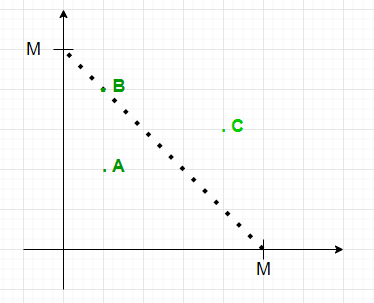Open in App
Not now

# Queries to count points lying on or inside an isosceles Triangle with given length of equal sides

• Last Updated : 01 Jul, 2021

Given an array arr[][] of dimension N * 2 representing the co-ordinates of N points and an array Q[] consisting of M integers, the task for every element in the Q[i] is to find the number of points lying inside or on the right-angled isosceles triangle formed on the positive co-ordinate axis with two equal sides of length Q[i]in each query.

Examples:

Input: N = 4, arr[][] = { {2.1, 3.0}, {3.7, 1.2}, {1.5, 6.5}, {1.2, 0.0} }, Q[] = { 2, 8, 5}, M = 3
Output: 1 4 2
Explanation:

• First query: The point (1.2, 0.0) lies inside the triangle.
• Second query: The points { (2.1, 3.0), (3.7, 1.2), (1.2, 0.0) } lies inside the triangle and point (1.5, 6.5) lies on the triangle.
• Third query: The points {(3.7, 1.2), (1.2, 0.0)} lies inside the triangle.

Input: N = 3, arr[][] = { {0, 0}, {1, 1}, {2, 1} }, Q[] = {1, 2, 3}, M = 3
Output: 1 2 3
Explanation:

• First query: The point (0, 0) lies inside the triangle.
• Second query: The points { (0, 0), (1, 1) } lies inside the triangle.
• Third query: All the points lies inside the triangle.

Naive Approach: The simplest approach is to in each query traverse the array of points and checks if it lies inside the right-angled triangle formed. After completing the above steps, print the count.
Time Complexity: O(N * M)
Auxiliary Space: O(1)

Efficient Approach: The above approach can be optimized based on the following observations:• A point (x, y) lies inside the isosceles right angle triangle formed on co-ordinate axis whose two equal sides are X if :
• x ≥ 0 && y ≥ 0 && x + y ≤ X
• By pre-storing the count of points with coordinates sum ≤ X, the query can be answered in constant time.

Follow the steps below to solve the problem:

Below is the implementation of the above approach:

## C++

 `// C++ implementation of above approach` `#include ``using` `namespace` `std;` `int` `const` `MAX = 1e6 + 5;` `// Function to find answer of each query``int` `query(vector > arr,``          ``vector<``int``> Q)``{``    ``// Stores the count of points with sum``    ``// less than or equal to their indices``    ``int` `pre[MAX] = { 0 };` `    ``// Traverse the array``    ``for` `(``int` `i = 0; i < arr.size(); i++) {` `        ``// If both x and y-coordinate < 0``        ``if` `(arr[i] < 0 || arr[i] < 0)``            ``continue``;` `        ``// Stores the sum of co-ordinates``        ``int` `sum = ``ceil``((arr[i] + arr[i]));` `        ``// Increment count of sum by 1``        ``pre[sum]++;``    ``}` `    ``// Prefix array``    ``for` `(``int` `i = 1; i < MAX; i++)``        ``pre[i] += pre[i - 1];` `    ``// Perform queries``    ``for` `(``int` `i = 0; i < Q.size(); i++) {``        ``cout << pre[Q[i]] << ``" "``;``    ``}``    ``cout << endl;``}``// Drivers Code``int` `main()``{``    ``vector > arr = { { 2.1, 3.0 },``                                   ``{ 3.7, 1.2 },``                                   ``{ 1.5, 6.5 },``                                   ``{ 1.2, 0.0 } };``    ``vector<``int``> Q = { 2, 8, 5 };``    ``int` `N = arr.size();``    ``int` `M = Q.size();` `    ``query(arr, Q);``}`

## Java

 `// Java implementation of above approach``import` `java.util.*;``class` `GFG``{` `static` `int` `MAX = (``int``) (1e6 + ``5``);` `// Function to find answer of each query``static` `void` `query(``double` `[][]arr,``          ``int` `[]Q)``{``  ` `    ``// Stores the count of points with sum``    ``// less than or equal to their indices``    ``int` `pre[] = ``new` `int``[MAX];` `    ``// Traverse the array``    ``for` `(``int` `i = ``0``; i < arr.length; i++)``    ``{` `        ``// If both x and y-coordinate < 0``        ``if` `(arr[i][``0``] < ``0` `|| arr[i][``1``] < ``0``)``            ``continue``;` `        ``// Stores the sum of co-ordinates``        ``int` `sum = (``int``) Math.ceil((arr[i][``0``] + arr[i][``1``]));` `        ``// Increment count of sum by 1``        ``pre[sum]++;``    ``}` `    ``// Prefix array``    ``for` `(``int` `i = ``1``; i < MAX; i++)``        ``pre[i] += pre[i - ``1``];` `    ``// Perform queries``    ``for` `(``int` `i = ``0``; i < Q.length; i++)``    ``{``        ``System.out.print(pre[Q[i]]+ ``" "``);``    ``}``    ``System.out.println();``}``  ` `// Drivers Code``public` `static` `void` `main(String[] args)``{``    ``double``[][] arr = { { ``2.1``, ``3.0` `},``                                   ``{ ``3.7``, ``1.2` `},``                                   ``{ ``1.5``, ``6.5` `},``                                   ``{ ``1.2``, ``0.0` `} };``    ``int` `[]Q = { ``2``, ``8``, ``5` `};``    ``int` `N = arr.length;``    ``int` `M = Q.length;` `    ``query(arr, Q);``}``}` `// This code is contributed by 29AjayKumar`

## Python3

 `# Python3 implementation of above approach``MAX` `=` `10``*``*``6` `+` `5``from` `math ``import` `ceil` `# Function to find answer of each query``def` `query(arr, Q):``  ` `    ``# Stores the count of points with sum``    ``# less than or equal to their indices``    ``pre ``=` `[``0``]``*``(``MAX``)` `    ``# Traverse the array``    ``for` `i ``in` `range``(``len``(arr)):` `        ``#` If both x and y-coordinate < 0``        ``if` `(arr[i][``0``] < ``0` `or` `arr[i][``1``] < ``0``):``            ``continue` `        ``# Stores the sum of co-ordinates``        ``sum` `=` `ceil((arr[i][``0``] ``+` `arr[i][``1``]));` `        ``# Increment count of sum by 1``        ``pre[``sum``] ``+``=` `1` `    ``# Prefix array``    ``for` `i ``in` `range``(``1``, ``MAX``):``        ``pre[i] ``+``=` `pre[i ``-` `1``]` `    ``# Perform queries``    ``for` `i ``in` `range``(``len``(Q)):``        ``print``(pre[Q[i]], end ``=` `" "``)``        ` `# Drivers Code``if` `__name__ ``=``=` `'__main__'``:``    ``arr ``=` `[[ ``2.1``, ``3.0``],``          ``[ ``3.7``, ``1.2``],``          ``[ ``1.5``, ``6.5``],``          ``[ ``1.2``, ``0.0``]]``    ``Q ``=` `[``2``, ``8``, ``5``]``    ``N ``=` `len``(arr)``    ``M ``=` `len``(Q)` `    ``query(arr, Q)` `    ``# This code is contributed by mohit kumar 29.`

## C#

 `// C# implementation of above approach``using` `System;``public` `class` `GFG``{` `  ``static` `int` `MAX = (``int``) (1e6 + 5);` `  ``// Function to find answer of each query``  ``static` `void` `query(``double` `[,]arr,``                    ``int` `[]Q)``  ``{` `    ``// Stores the count of points with sum``    ``// less than or equal to their indices``    ``int` `[]pre = ``new` `int``[MAX];` `    ``// Traverse the array``    ``for` `(``int` `i = 0; i < arr.GetLength(0); i++)``    ``{` `      ``// If both x and y-coordinate < 0``      ``if` `(arr[i,0] < 0 || arr[i,1] < 0)``        ``continue``;` `      ``// Stores the sum of co-ordinates``      ``int` `sum = (``int``) Math.Ceiling((arr[i,0] + arr[i,1]));` `      ``// Increment count of sum by 1``      ``pre[sum]++;``    ``}` `    ``// Prefix array``    ``for` `(``int` `i = 1; i < MAX; i++)``      ``pre[i] += pre[i - 1];` `    ``// Perform queries``    ``for` `(``int` `i = 0; i < Q.Length; i++)``    ``{``      ``Console.Write(pre[Q[i]]+ ``" "``);``    ``}``    ``Console.WriteLine();``  ``}` `  ``// Drivers Code``  ``public` `static` `void` `Main(String[] args)``  ``{``    ``double``[,] arr = { { 2.1, 3.0 },``                     ``{ 3.7, 1.2 },``                     ``{ 1.5, 6.5 },``                     ``{ 1.2, 0.0 } };``    ``int` `[]Q = { 2, 8, 5 };``    ``int` `N = arr.GetLength(0);``    ``int` `M = Q.Length;``    ``query(arr, Q);``  ``}``}` `// This code is contributed by 29AjayKumar`

## Javascript

 ``

Output:

`1 4 2`

Time Complexity: O(N)
Auxiliary Space: O(N)

My Personal Notes arrow_drop_up